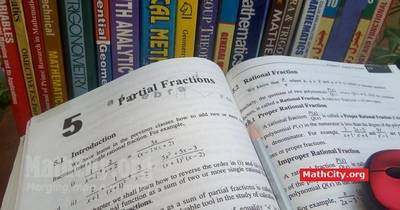# Chapter 05: Partial FractionsNotes (Solutions) of Chapter 05: Partial Fractions, Text Book of Algebra and Trigonometry Class XI (Mathematics FSc Part 1 or HSSC-I), Punjab Text Book Board, Lahore.

• Introduction
• Rational Fraction
• Proper Rational Fraction
• Improper Rational Fraction
• Resolution of a Rational Fraction $\frac {P(x)}{Q(x)}$ into Partial Fractions
• Exercise 5.1
• Exercise 5.2
• Exercise 5.3
• Exercise 5.4

The following short questions was send by Mr. Akhtar Abbas.

• fsc/fsc_part_1_solutions/ch05